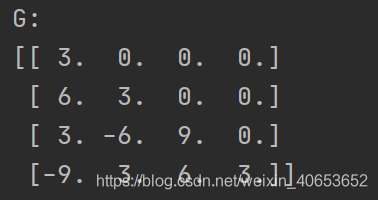# Python’s direct method for solving linear equations (5) — square root method for solving linear equations

The square root method solves systems of linear equations

``````import numpy as np

def pingfagenfa(a):
n = a.shape
g = np.mat(np.zeros((n, n), dtype=float))
g[0, 0] = np.sqrt(a[0, 0])
g[1:, 0] = a[1:, 0]/g[0, 0]
for j in range(1, n-1):
g[j, j] = np.sqrt((a[j, j] - np.sum(np.multiply(g[j, 0:j], g[j, 0:j]))))
for i in range(j+1, n):
g[i, j] = (a[i, j] - np.sum(np.multiply(g[i, 0:j], g[j, 0:j])))/g[j, j]
g[n-1, n-1] = np.sqrt((a[n-1, n-1] - np.sum(np.multiply(g[n-1, 0:n-1], g[n-1, 0:n-1]))))
return g

if __name__ == "__main__":
a = np.mat([[9, 18, 9, -27],
[18, 45, 0, -45],
[9, 0, 126, 9],
[-27, -45, 9, 135]], dtype=float)
print('G:')
G = pingfagenfa(a)
print(G)
``````

The solution results are shown in the figure below:column
Python is the direct method of solving linear equations (1) — – gaussian elimination
Python the direct method of solving linear equations (2) — – gaussian column main element elimination
Python the direct method of solving linear equations (3) — – column if primary element gauss – when elimination
Python the direct method of solving linear equations (4) — – LU decomposition of the matrix
Python the direct method of solving linear equations (5) — –
square root method to solve linear equations Python direct method for solving systems of linear equations (6) — chase method for solving tridiagonal equations
Python direct method for solving systems of linear equations (7) — givens transformation based QR decomposition
Python direct method for solving systems of linear equations (8) — haushalde transformation based QR decomposition